Journal of Water Resources and Ocean Science
Volume 5, Issue 6, December 2016, Pages: 87-92

A Comparison Study of Seawater Dissolved Oxygen Using Dynamic Changes Prediction Models

Wang Cao1, 2, Jingya Sun1, M. V. Subrahmanyam1, Feng Gui1, *

1Department of Marine Science and Technology, Zhejiang Ocean University, Zhoushan, , China

2Marine Biology Institute, Shantou University, Shantou, China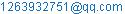(Wang Cao)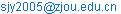(Jingya Sun)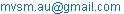(M. V. Subrahmanyam)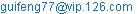(Feng Gui)

*Corresponding author

Wang Cao, Jingya Sun, M. V. Subrahmanyam, Feng Gui. A Comparison Study of Seawater Dissolved Oxygen Using Dynamic Changes Prediction Models. Journal of Water Resources and Ocean Science. Vol. 5, No. 6, 2016, pp. 87-92.doi: 10.11648/j.wros.20160506.12

Received: October 11, 2016; Accepted: October 20, 2016; Published: November 18, 2016

Abstract: Dissolved oxygen (DO) is a key water quality parameter and dynamic change prediction of water quality can provide a necessary assistance to solve the marine pollution problem. In this study, DO concentration data were collected from the buoy near Aoshan Island, Zhoushan, China. Based on DO concentration analysis, three prediction model were established, which includes Grey prediction model (GM (1,1)), back propagation(BP) neural network prediction model and the combination of GM-BP neural network prediction model. All three models have high fitting degree and the average relative error for each model is 9.1482%, 1.8940% and 0.2195% respectively. Hence, the combination of GM-BP neural network prediction model has highest accuracy than BP neural network prediction modeland GM (1,1) prediction model.Combination of prediction model has more advantages than a single prediction model and it is possible to improve the accuracy of prediction for better results.

Keywords: Dissolved Oxygen, Dynamic Change Prediction, GM (1,1) Prediction Model, BP Neural Network, Combination Prediction Model

Contents

1. Introduction

The Marine Environment Bulletin data was indicated that large areas of China’s coastal waters were under an unhealthy statefor several years.However, besides the regular marine monitoring data, dynamic change prediction of water quality can provide a necessary assistance to solve the marine pollution problem. In recent years, several studies have been carried out worldwide on water quality prediction models to provide the necessary theoretical basis for marine environment protection.

Traditional water quality prediction models include water quality simulation, historical valuation method, linear regression, climatologically mean, the gray prediction (Julong 1989)and so on. These methods cannot have high precision and fitness when dealing with uncertain fuzzy dynamic changes of water quality.Artificial neural network (ANN) hasfour characteristics-nonlinear, non-limiting, high qualitative and non-convexity. ANN is powerful adaptive, self-organizing, self-learning ability and the ability of infinite nonlinear function approximation. Also ANN is a powerful tool to handle and excavate data relationships and establish prediction model.

In recent years, several scientists have been working on prediction of water quality especially in China. Byestablished a reasonable low flow backpropagation (BP) neural network prediction model, which has a high degree of fitness and improved accuracy (Sun et al2004). Based on BP neural network, which was used levenberg-marquard (LM) algorithm, predicted the water quality of Qiantang River with a maximum error of 11.7% and the mean error of 4.3% (Wang et al2007). In addition, based on the limitation of traditional neural networks,a new neural network model was proposed by correcting the artificial neural network weight algorithm, optimizeneural network structure and global convergence algorithm (Yu et al 2011). Since Bates and Granger (1969) proposed a combined prediction model for the first time.Several researchers pointed out that a combination of two or more prediction models can increase the performance, because different prediction models have their own independent systems information. Several researchers applied ANN to water quality prediction, established a gray neural network prediction model (Ju et al 2007, Guo et al 2015, Zhou and Zhou 2011), which used a method of optimum weights. Results reveal that the combined prediction model performance is better than a single prediction model. By design a combined prediction model used weighted algorithm andthe resultindicating that the combination prediction model is superior to single model(Wang 2016). However, when a single nonlinear model is forecasted by single prediction method or condition desired signal based on the information collected is a nonlinear function, the respective prediction method’s linear combination is not optimal (Si 1998). Obtain a real-coded accelerate genetic algorithm (RAGA), which is improved grey BP neural network prediction model by using gray prediction results to neural network as training and obtained relatively high accuracy in prediction(Liu et al2008).

In this paper, dissolved oxygen (DO) concentration data were used to predict DO dynamic changes by using different prediction models. We apply the residuals ofGM (1,1) prediction model as an input value for BP neural network to train in-depth combination process (nonlinear combination). The combination of GM-BP neural network prediction model established to improve the prediction accuracy of the prediction and process. Comparison study has been done between the single GM (1,1) prediction model and BP neural network prediction model, in order to provide a reliable forecast information for the marine water environment management.

2. Data and methodology

2.1. Data Sources and Preprocessing

It is worth mentioning that the source of real-time Monitoring DO sate is the marine buoy near Aoshan Island. The data collected from 2013.08.20 to 2015.04.30 with sampling period of 15minutes.

Preprocessing of original data was filtered and averaged, and 504 training sample data were obtained for modeling. Sample of data was given in the Table 1. The period of data chosen as input for the model was between 2015.04.21 and 2015.04.30.

Table 1.Training of sample data.

 time DO(mg/l) time DO(mg/l) time DO(mg/l) 2013/8/20 5.3289 2013/10/11 6.4252 2014/10/15 6.3918 2013/8/21 5.4200 2013/10/12 6.4239 2014/10/16 6.4005 …… …… …… …… …… …… 2013/10/8 6.5450 2014/11/13 7.0706 2015/4/19 8.5514 2013/10/10 6.4823 2014/11/14 7.1110 2015/4/20 8.5370

2.2. Introduction to Three Prediction Models

2.2.1. GM (1,1) Prediction Model

This study mainly based on the Grey Series Forecastingtheory.The Algorithm principle and modeling methodof GM (1,1) prediction model were describes as followed:

1) Once accumulated generating

Primary data series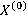: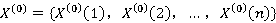, whereis generated by once accumulated generating sequence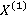: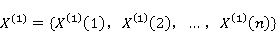(1)

Where,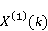=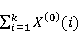k=12n,  (2)

2) Background value sequence construction

Using once accumulated generating sequence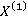to generate the background value sequence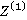,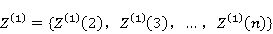(3)

Where,=k=12n,   (4)

3) Establishing albino equation

Using once accumulated generating sequenceto establish albino equation,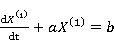(5)

Discredited the above formula, and get GM (1,1) gray differential equation: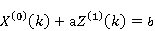(6)

Whereas the development factor, b is the amount of gray effect.

4) Calculation of Parameters

Using the least squares method, we can solve the parameters (a and b) in the formula (6), namely: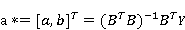(7)

Where,,        (8)(9)

5) Solvingalbino equation and grey differential equation, and get gray GM (1,1) Prediction Model

Where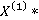and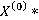as the predicted value ofand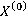, and by solve albino equation and grey differential equation, we get: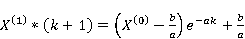k=12n,  (10)

Then after a regressive reduction, we get: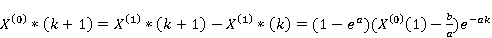k=12n,  (11)

2.2.2. BP neural Network Prediction Model

Artificial Neural Networkis a mathematical modelto mimic animalneural network behavior and characteristics and distributeparallel information processing. At the middle of last century,BP neural network algorithm was firstestablishedandapplied, which was a multi-layer feed forward network by usingthe back propagation algorithm for network training(David et al 1986). BP neural network consists of two parts, whichwere information dissemination and forward error back propagation. BP neural network is multi-layer network architecture, consists three parts: an input layer, a hidden layer and an output layer. Each input layer neurons receive outside information (data) and passed to the hidden layer (middle layer), which can be considered as aninformation processing layer. Hidden layer designed that theinformation wouldtransmit to the output layer.Then to the next processing treatment layer, the forward end of a propagation process.While the output of information processing results to the outside world by the output layer. If the outputs and the desired output errors could not meet the requirements, the error will be fed back to the output layer, and the layerweightswill be corrected according to the error gradient descent way, and then be passed to the input layer and the hidden layeruntil training modes can meet the requirements.

For practical applications, the traditional forms of BP neural network can hardly achieve the desired effect, need to improve the algorithm. The improved BP neural networkthat was used LM algorithmto predictwater quality, and gota better accuracy and reliability degree (Tian et al2010). LMalgorithm was used for this study.

2.2.3.Combinationof GM-BP Neural network Prediction Model

Figure 1explainsthe overall framework of combination of GM-BP neural network prediction model, and the specific steps are follows:

1) to establish GM (1,1) prediction model using the original data series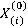, process accumulation and get a cumulative sequence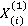by GM (1,1) prediction model and establish the albino equation and gray differential equation, then get the fitting data sequence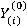ofGM (1,1) prediction model;

2) Calculate the GM (1,1) model’s residuals data sequence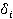,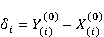(12)

3) Usingthe GM (1,1) prediction model to obtain a gray prediction series;

4) Using BP neural network modelto train GM (1,1) model residuals data sequenceand process for reasonable prediction, obtain residual (BP) prediction series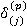;

5) According to the relationship between the gray prediction and residual prediction results: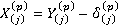,to obtain a combination of the model prediction series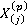.

Whena single nonlinear model forecasted by a single prediction method or condition desired signal based on the information collected from a nonlinear function.Neitherprediction methods nor linear combination is optimal, so chosen the GM (1,1) prediction model residuals as BP neural network training data to establish networking.Then combination of depth and establish a combination of GM-BP neural network prediction model, which can improve the accuracy of prediction model.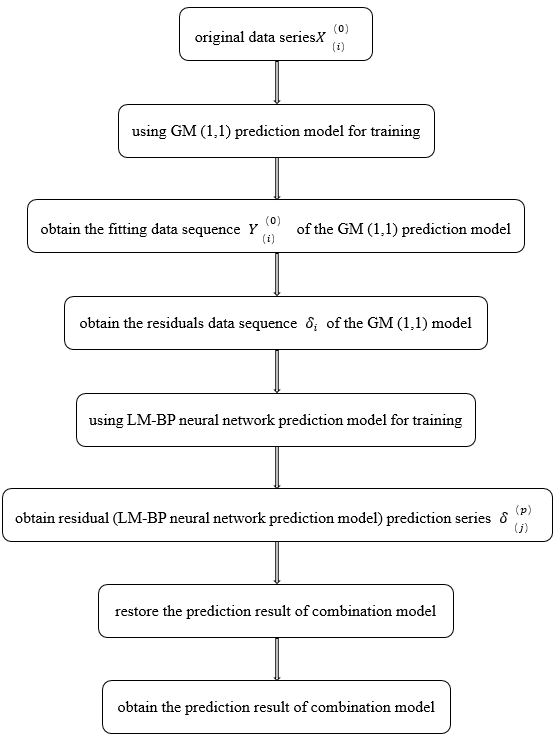Figure 1. The combination of GM-BP neural network prediction model.

3. Case study

Using the three modelsmentioned above, GM (1,1) prediction model, LM-BP network prediction model and combination of GM-BP neural network prediction model, the DO concentration of AoShan sea area were predicted. The modeling data sequences were from2013.08.20 to 2015.04.20.

3.1. GM (1,1) Prediction Model

Sample data of DO concentration over Aoshan sea area from2013.08.20 to 2015.04.20 were selected as the training sample data.Equal dimensionrecurrence GM (1,1) forecast model was used to (see Section 1.2 specific principles) establish prediction model and predictthe following tendaysand the results were illustratedin table2and table3.

3.2. BP neural Network Prediction Model

Select a three layers BP neural network prediction model, including one hidden layer. The BP neural network parameter, include that learning algorithm isLM algorithm,transfer function of the input layer and the hidden layer is sigmoid type function, the output layer function is linear transfer function, train 10,000 steps, learning rate is 0.05, prediction accuracy is 0.0001, prediction length is 10, hidden layer neuron is 8 (drawn from the experience). Finely, 504 sample data of DO concentration in Aoshan sea area from 2013.08.20 to 2015.04.20 as the training data. In order to improve the speed of network convergence, the training data were normalized and converted to [0,1], to build the training network. Training results were given in table2 and table3.

3.3. Combination of GM-BP Neural Network Prediction Model

Selected 504 sample data of DO concentration in Aoshan sea area from2013.08.20 to 2015.04.20 were used as the training data, by GM (1,1) prediction model obtain the fits data sequenceand residualssequence, use BP neural network model network trainthe residual series, and obtain residual prediction sequence.Finally, according to the relationship between the gray prediction and residual prediction results: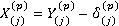, obtain the combination model prediction series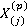. The results were given in table2and table3.

Table 2. The prediction results of the three prediction models.

 Time actual value(mg/L) Predictive value(mg/L) GM prediction model BP neural network prediction model Combinationof GM-BP neural network prediction model 2015/4/21 8.5233 7.6178 8.5401 8.5051 2015/4/22 8.4965 7.6182 8.5412 8.4799 2015/4/23 8.4684 7.6187 8.5431 8.4534 2015/4/24 8.4440 7.6192 8.5448 8.4257 2015/4/25 8.4197 7.6197 8.5463 8.3967 2015/4/26 8.3862 7.6202 8.5475 8.3663 2015/4/27 8.3536 7.6207 8.5484 8.3346 2015/4/28 8.3247 7.6212 8.5492 8.3016 2015/4/29 8.2393 7.6217 8.5498 8.2672 2015/4/30 8.2283 7.6222 8.5503 8.2316

Table 3. The results of the relative error of the three prediction model.

 Time The absolute value of the relative error(%) GM prediction model BP neural networkprediction model combination of GM-BP neural network prediction model 2015/4/21 10.6242 0.1973 0.2135 2015/4/22 10.3363 0.5267 0.1954 2015/4/23 10.0331 0.8820 0.1766 2015/4/24 9.7673 1.1944 0.2160 2015/4/25 9.5009 1.5042 0.2725 2015/4/26 9.1340 1.9233 0.2368 2015/4/27 8.7732 2.3328 0.2267 2015/4/28 8.4506 2.6971 0.2773 2015/4/29 7.4964 3.7682 0.3387 2015/4/30 7.3659 3.9138 0.0413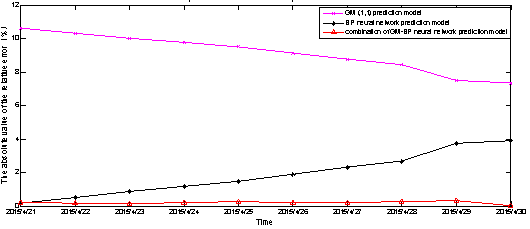Figure 2. The prediction results of the three prediction models.

4. Results and Discussion

Figure2indicates the relative errordiagram of three predictions models.It is very intuitivethat the relative error of combination of GM-BP neural network prediction model (red line) is significantly lower, howeverother two single prediction models are predicting with higher error. In addition, the prediction resultsare presented in table 2and the relative errors givenin table 3. When the three prediction modelscompared, the result of combination of GM-BP neural network prediction model is close to the true value.

There indexes, maximum, minimum and average relative error relative error were selected to comparethe accuracy of the three models. As shown in table 4, GM (1,1) prediction model has the maximum relative error of 10.6242%, the minimum relative error is 7.3659% and the average relative error was 9.1482%; BP neural network prediction model works with the maximum relative error of 3.9138%, the minimum relative error is 0.1973% and the average relative error was 1.8940%; Combination of GM-BP neural network prediction model predicts have the best performance, with the maximum relative error is 0.3387%, the minimum relative erroris0.0413% andthe average relative error is 0.2195%.

Prediction error variance of three predictionsmodel (GM (1,1) prediction model, BP neural network prediction model and the combination of GM-BP neural network prediction model) were 0.0096, 0.01 and 0.0002 respectively. Figure3depicts the error variance of combination of GM-BP neural network prediction model outperformedany single prediction model.

The above data also reveals that thecombination of GM-BP neural network prediction model is better than a single GM (1,1) prediction model and BP neural network prediction model.Therefore, combination model has a certain advantage for prediction of DO in seawater model.

Table 4. Comparethe prediction accuracy of the three models.

 relative error GM prediction model BP neural network prediction model combination of GM-BP neural network prediction model maximum 10.6242% 3.9138% 0.3387% minimum 7.3659% 0.1973% 0.0413% average 9.1482% 1.8940% 0.2195%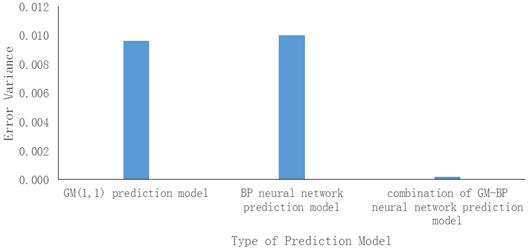Figure 3. Comparison of error variance of three prediction models.

Combination model compared with the prediction of a single model has an obvious advantagein the oceanDO, but the external environmental factors (such as: temperature, pH, salinity, nutrients) also having a great influence on DOvariations. Hence,by established a TS fuzzy neural network integrated model to give a higher accuratepre-warning methodsof water quality and found relationship of each single factor in the study over three Taihu Lake Basin(Zhang and Gao 2015).This paper only considered the relationship between the time seriesof DOso that the model has some limitations, and establish a multi-factor model prediction will be more reasonable.

5. Conclusions

Dynamic change prediction of water quality can provide important information for the pre-warning for oceans and marine protection. This paper uses GM (1,1) prediction model, BP neural network prediction model and combination of GM-BP neural network combination prediction model.Comparedthe three prediction models and results are as follows:

(1) The prediction accuracy of combination of GM-BP neural network prediction model is the higher than BP network prediction model and GM (1,1) prediction model, which isleast.

(2) GM (1,1) prediction model can handle the samplewithsmaller data set, andits discrete data fitting is poor.

(3) LM-BP neural network use the least squares method, which can effectively solve the traditional BP neural network exist easily falling into local minima and improve the accuracy of the model;

(4) GM-BP neural network combination prediction modelhaving the advantages of both models because usethe GM (1,1) prediction model residuals as BP (LM algorithm) neural network training sample, improves the prediction accuracy of water quality.

Acknowledgement

This work was supported by special research grant for non-profit public service, National Bureau of Oceanography (201305012-2).

References

1. Julong D. Introduction to grey system theory. The Journal of grey system, 1989, 1(1): 1-24.
2. Sun Shijun, Li Rui, Xie Xinmin, Liu Zuoxin. Application of BP network in real-time forecast of drought period surface runoff in guanyinge reservoir of Liaoning. Journal of Shenyang Agricultural University, 2004, 35(5-6):504-506
3. Wang Xiaoping, Sun Jiyang, Jin Xin. Prediction of water quality index in Qiantang River based on BP neural network model. Journal of Zhejiang University(Engineering Science), 2007, 41(2): 361-364.
4. Yu Yingjie, Jiang Weigang, Xu Mingfang. Prediction of chlorophyll a by BP neural network model based on PSO algorithm. Research of environmental sciences, 2011, 24(5): 526-532.
5. Bates J M, Granger C W J. The combination of forecasts. Journal of the Operational Research Society, 1969, 20(4): 451-468.
6. Ju Qin, Hao Zhenchun, Liu Jie. Prediction of river quality using the united gray neural network model. Journal of Hebei University of Engineering(Natural Science Edition), 2007, 24(3): 26-28.
7. Guo Lanlan, Zou Zhihong, An Yan. Study on grey model combined with artificial neural networks model for water quality forecast. Mathematics in practice and theory, 2015 (5): 89-93.
8. Zhou Bo, Zhou Hui. Center approach grey BP neural network prediction model for water quality. Haihe water resources, 2011 (6): 34-37.
9. Wang Jian. Research on prediction of water resource based on improved combination neural network. Computer Science. 2016, 1.
10. Si Xin. Neural network model in method of prediction. Forecasting, 1998, 2: 32-35.
11. Liu Rentao, Fu Qiang, Feng Yan, Gai Zhaomei, Li Guoliang, Li Weiye. Grey BP neural networks model based on RAGA and its application in groundwater dynamic prediction of the Sanjiang plain. System Engineering Theory and Practice, 2008, 28(5): 171-176.
12. David E. Rumelhart, Geoffrey E. Hinton, and Ronald J. Williams. Learning representations by back-propagating errors. Nature, 1986, 323: 533-536.
13. Tian Jianping, Cao Dongwei, Li Hainan. Application of LM-BP Neural Network in Water Quality Prediction for Yu Qiao Reservoir. Water resources in formatization, 2010 (3): 31-34.
14. Zhang Ying, Gao Qianqian. Comprehensive prediction model of water quality based on Grey Model and Fuzzy Neural Network. Chinese Journal of environmental Engineering, 2015 (2): 537-545.

 Contents 1. 2. 2.1. 2.2. 3. 3.1. 3.2. 3.3. 4. 5.
Article ToolsAbstractPDF(697K)